Next: Terrestrial Ocean Tides Up: Waves in Incompressible Fluids Previous: Wind Driven Waves in

# Exercises

1. Find the velocity potential of a standing gravity wave in deep water for which the associated elevation of the free surface is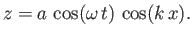Determine the paths of water particles perturbed by the wave.
2. Deep water fills a rectangular tank of lengthand breadth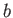. Show that the resonant frequencies of the water in the tank are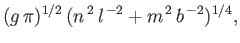where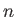andare integers. Neglect surface tension.

3. Demonstrate that a sinusoidal gravity wave on deep water with surface elevation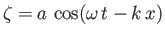possesses a mean momentum per unit surface area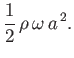4. A seismic wave passes along the bed of an ocean of uniform depthsuch that the vertical perturbation of the bed is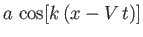. Show that the amplitude of the consequent gravity waves at the surface is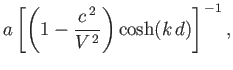where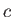is the phase velocity of waves of wavenumber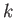.

5. A layer of liquid of densityand depthhas a free upper surface, and lies over liquid of infinite depth and density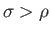. Neglecting surface tension, show that two possible types of wave of wavenumber, with phase velocities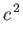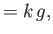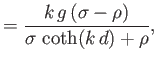can propagate along the layer.

6. Show that, taking surface tension into account, a sinusoidal wave of wavenumberand surface amplitude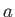has a mean kinetic energy per unit surface area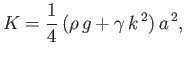and a mean potential energy per unit surface area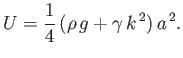7. Show that in water of uniform depththe phase velocity of surface waves can only attain a stationary (i.e., maximum or minimum) value as a function of wavenumber,, when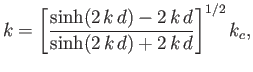where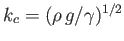. Hence, deduce that the phase velocity has just one stationary value (a minimum) for any depth greater than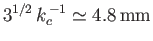, but no stationary values for depths less than that.

8. Unlike gravity waves in deep water, whose group velocities are half their phase velocities, the group velocities of capillary waves aretimes their phase velocities. Adapt the analysis of Section 11.7 to investigate the generation of capillary waves by a very small object traveling across the surface of the water at the constant speed. Suppose that the unperturbed surface corresponds to the-plane. Let the object travel in the minus-direction, such that it is instantaneously found at the origin. Find the present position of waves that were emitted, traveling at an angle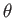to the object's direction of motion, when it was located at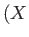,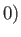. Show that along a given interference maximum the quantities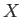andvary in such a manner that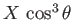takes a constant value,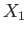(say). Deduce that the interference maximum is given parametrically by the equations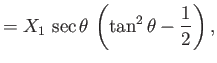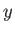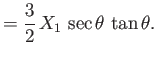Sketch this curve, noting that it goes through the points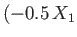,and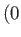,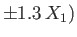, and asymptotes to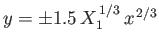.Next: Terrestrial Ocean Tides Up: Waves in Incompressible Fluids Previous: Wind Driven Waves in
Richard Fitzpatrick 2016-03-31